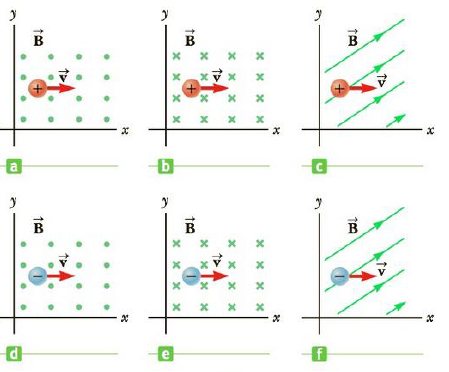# Figure CQ19.1 shows a positive and a negative charge each moving in the xy -plane through three different magnetic fields. In each case, find the direction of the magnetic force on the charged panicle. Indicate your answers as + x or − x for the positive or negative x - direction and similarly for the y - and z -directions. Figure CQ19.1### College Physics

11th Edition
Raymond A. Serway + 1 other
Publisher: Cengage Learning
ISBN: 9781305952300### College Physics

11th Edition
Raymond A. Serway + 1 other
Publisher: Cengage Learning
ISBN: 9781305952300

#### Solutions

Chapter
Section
Chapter 19, Problem 1CQ
Textbook Problem

## Figure CQ19.1 shows a positive and a negative charge each moving in the xy-plane through three different magnetic fields. In each case, find the direction of the magnetic force on the charged panicle. Indicate your answers as +x or −x for the positive or negative x- direction and similarly for the y- and z-directions.Figure CQ19.1

Expert Solution

a)

To determine
The direction of the magnetic force.

y

### Explanation of Solution

The magnetic force on a moving charged particle is given by,

F=q(v×B)

The velocity is directed along +x and the magnetic field is along +z axis. Hence using right hand thumb rule, the direction of the force will be y .

Conclusion: The direction of the magnetic force will be y .

Expert Solution

b)

To determine
The direction of the magnetic force.

+y

### Explanation of Solution

The magnetic force on a moving charged particle is given by,

F=q(v×B)

The velocity is directed along +x and the magnetic field is along z axis. Hence using right hand thumb rule, the direction of the force will be +y .

Conclusion: The direction of the magnetic force will be +y .

Expert Solution

c)

To determine
The direction of the magnetic force.

+z

### Explanation of Solution

The magnetic force on a moving charged particle is given by,

F=q(v×B)

The velocity is directed along +x and the magnetic field is in the xy plane. Hence using right hand thumb rule, the direction of the force will be +z .

Conclusion: The direction of the magnetic force will be +z .

Expert Solution

d)

To determine
The direction of the magnetic force.

y

### Explanation of Solution

The magnetic force on a moving negatively charged particle is given by,

F=q(v×B)

The velocity is directed along +x and the magnetic field is along +z axis. Hence using right hand thumb rule, the direction of the force will be +y .

Conclusion: The direction of the magnetic force will be y .

Expert Solution

e)

To determine
The direction of the magnetic force.

y

### Explanation of Solution

The magnetic force on a moving negatively charged particle is given by,

F=q(v×B)

The velocity is directed along +x and the magnetic field is along +z axis. Hence using right hand thumb rule, the direction of the force will be +y .

Conclusion: The direction of the magnetic force will be y .

Expert Solution

f)

To determine
The direction of the magnetic force.

z

### Explanation of Solution

The magnetic force on a moving charged particle is given by,

F=q(v×B)

The velocity is directed along +x and the magnetic field is in the xy plane. Hence using right hand thumb rule, the direction of the force will be z .

Conclusion: The direction of the magnetic force will be z .

### Want to see more full solutions like this?

Subscribe now to access step-by-step solutions to millions of textbook problems written by subject matter experts!

Get Solutions

### Want to see more full solutions like this?

Subscribe now to access step-by-step solutions to millions of textbook problems written by subject matter experts!

Get Solutions